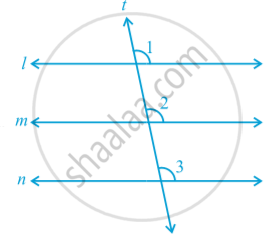Share

# Lines Parallel to the Same Line

#### notes

If two lines are parallel to the same line, will they be parallel to each other in following fig.Line m || line l and line n || line l.
Let us draw a line t transversal for the lines, l, m and n. It is given that line m || line l and line n || line l.
Therefore, ∠ 1 = ∠ 2 and ∠ 1 = ∠ 3
(Corresponding angles axiom)
So, ∠ 2 = ∠ 3
But ∠ 2 and ∠ 3 are corresponding angles and they are equal.
Therefore, you can say that
Line m || Line n
(Converse of corresponding angles axiom)

#### theorem

Theorem:  Lines which are parallel to the same line are parallel to each other.

Note : The property above can be extended to more than two lines also.
Now, let us solve some examples related to parallel lines.

### Shaalaa.com

Theorem: Lines which are parallel to the same line are parallel to each other. [00:09:59]
S
0%

S